Next: Shock Wave Up: Energy Equation Previous: Polytropic Relation   Contents

## Energy Equation from the First Law of Theromodynamics

In more general cases, the last equation comes from the first law of the thermal physics,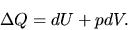(A.21)

where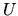and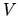represent the internal energy and the volume and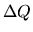is the heat added or subtracted from the system. Using the total energy per unit volume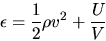(A.22)

the above equation gives the equation for total energy: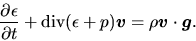(A.23)

Since the total energy per unit volume is expressed using the basic physical quantities as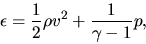(A.24)

equation (A.23) is the final basic equation for hydrodynamics. Equations (A.7), (A.11), and (A.23) are basic equations hydrodynamics using the Eulerian time derivative.

Problem: From the continuity equation (A.11) and the equation of motion (A.7) or the equation for momentum density (A.15), show that the equation for the kinetic enerygy density is expressed as follows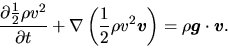(A.25)

Then, obtain equation for the total energy (A.23).Next: Shock Wave Up: Energy Equation Previous: Polytropic Relation   Contents
Kohji Tomisaka 2007-07-08